Question

# The one-line diagram of a three-bus power system is shown in Figure 4. All impedances are...

The one-line diagram of a three-bus power system is shown in Figure 4. All impedances are
expressed in per unit on a common MVA base. All resistances and shunt capacitances are
neglected. Information on each component in this system is given below:
• Each generator is represented by an emf
behind the sub-transient reactance of j0.045
and their neutrals are connected to the
ground.
• Line 1-2 has reactance of j0.88
• Line 2-3 has reactance of j0.65
• Line 1-3 has reactance of j0.4
• The generators are operating on no load at
their rated voltage (1.02 per unit) with their
emf in phase.
a) Construct the bus impedance matrix by adding one impedance element at a time and
using the matrix elimination technique, when needed.
b) Consider, a three-phase fault, which occur sequentially at bus 1, 2 and 3 through a fault
impedance of Zf=j0.20 per unit. Find the bus location at which fault current in per unit is
the most severe.
c) Determine the healthy bus voltages for the fault location at bus 3.
A second line is built between Bus 1 and Bus 2 with an impedance of j0.88.
d) Repeat Questions (a), (b) and (c) for the new power systems.
e) Compare the bus matrix in this case with the original bus matrix. What is the implication
of introducing the second transmission line between bus 1 and bus 2?
Figure 4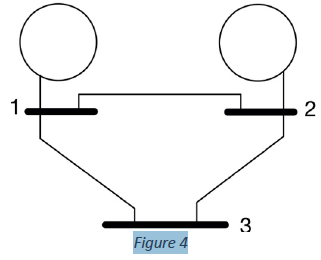Figure 4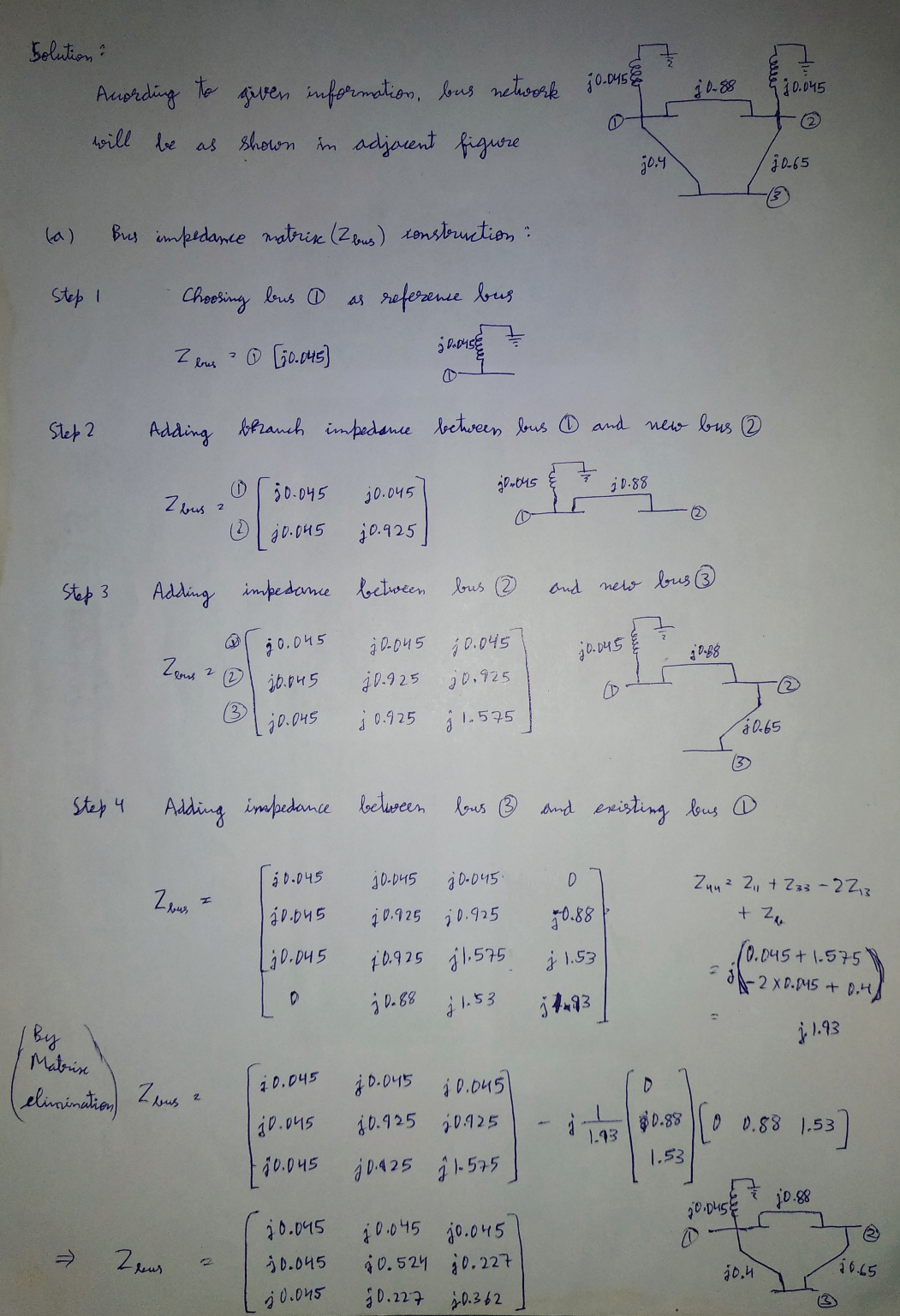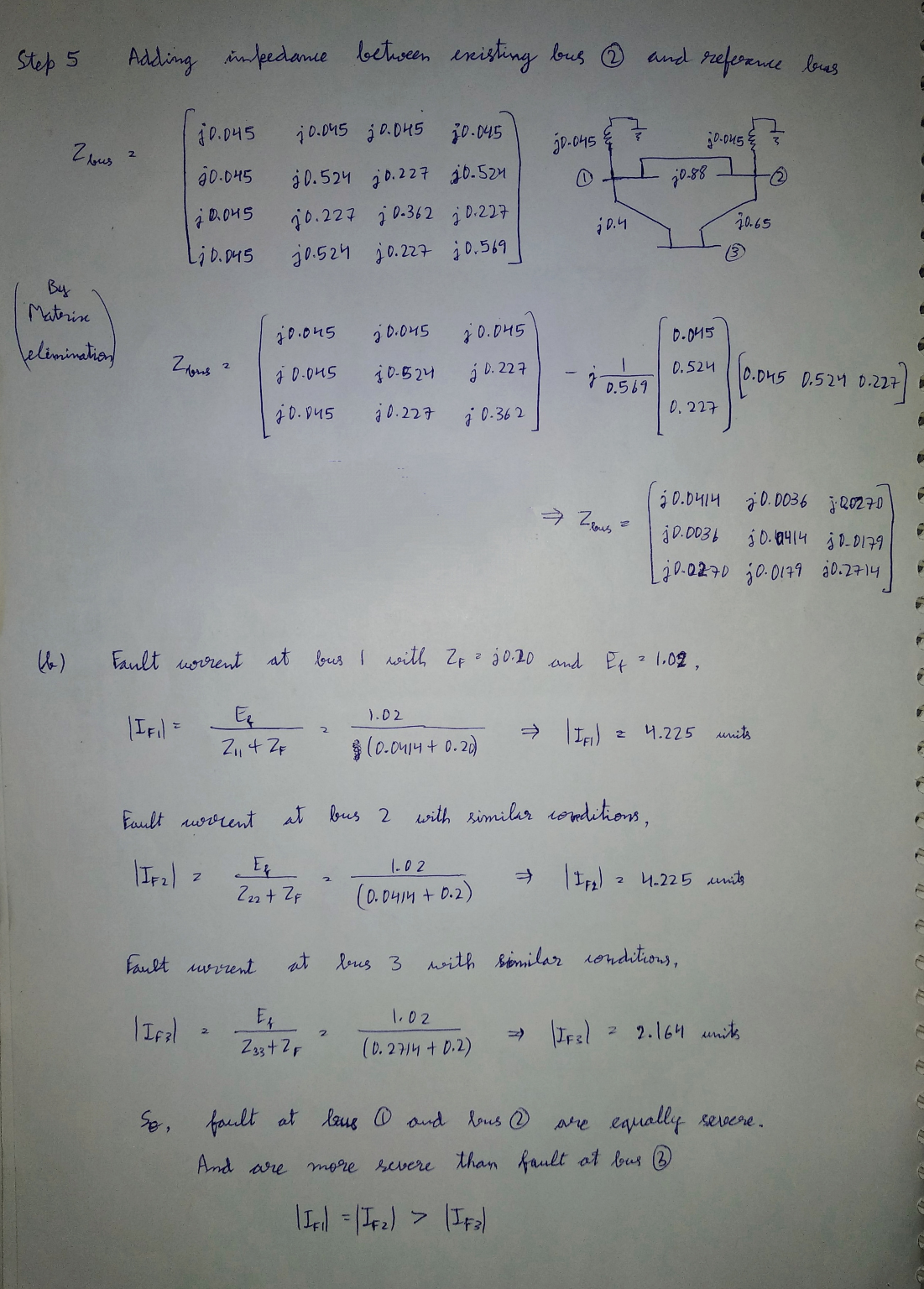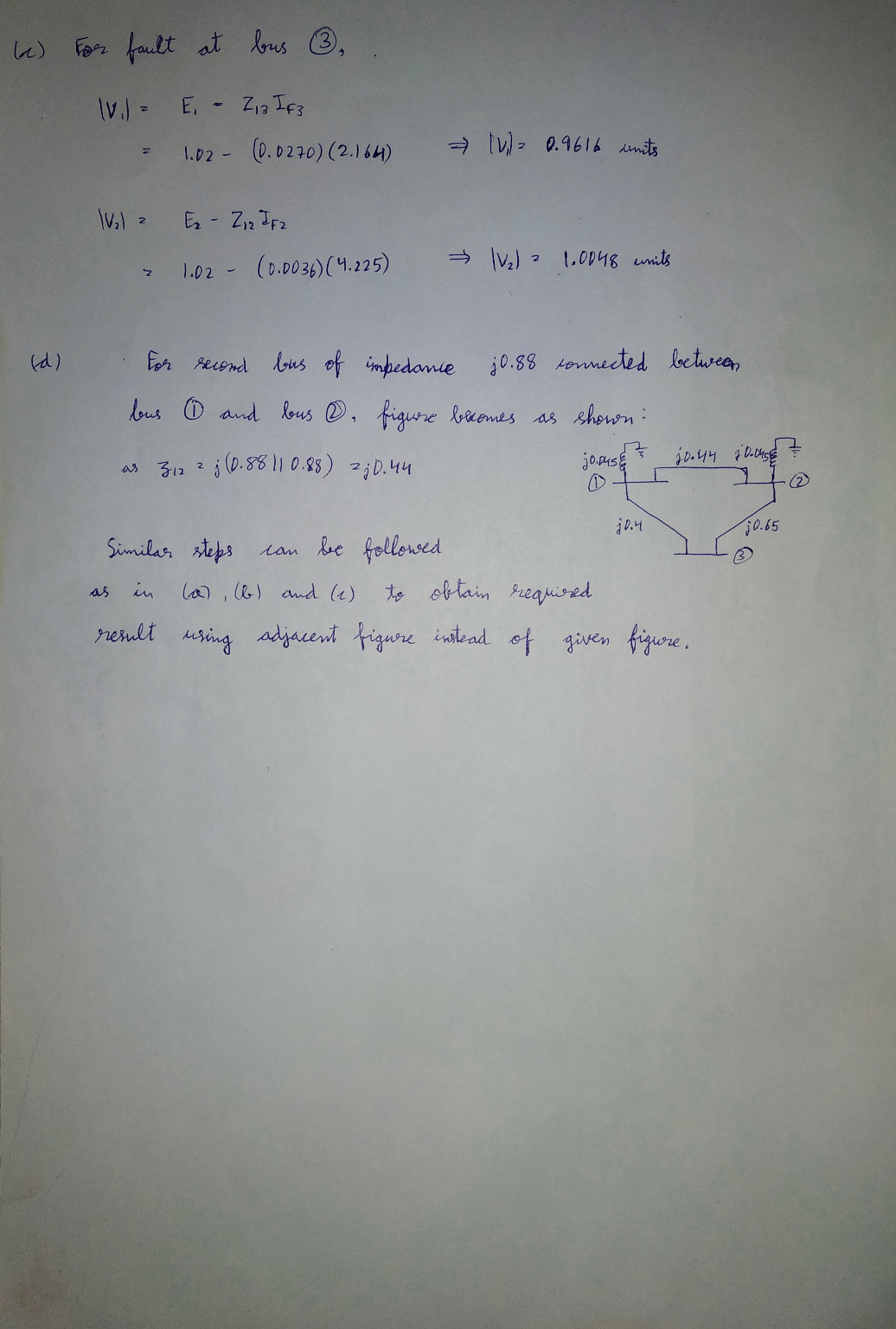#### Earn Coins

Coins can be redeemed for fabulous gifts.

Similar Homework Help Questions
• ### The one line diagram of a three-phase power system is shown in Fig.8. Impedances are marked...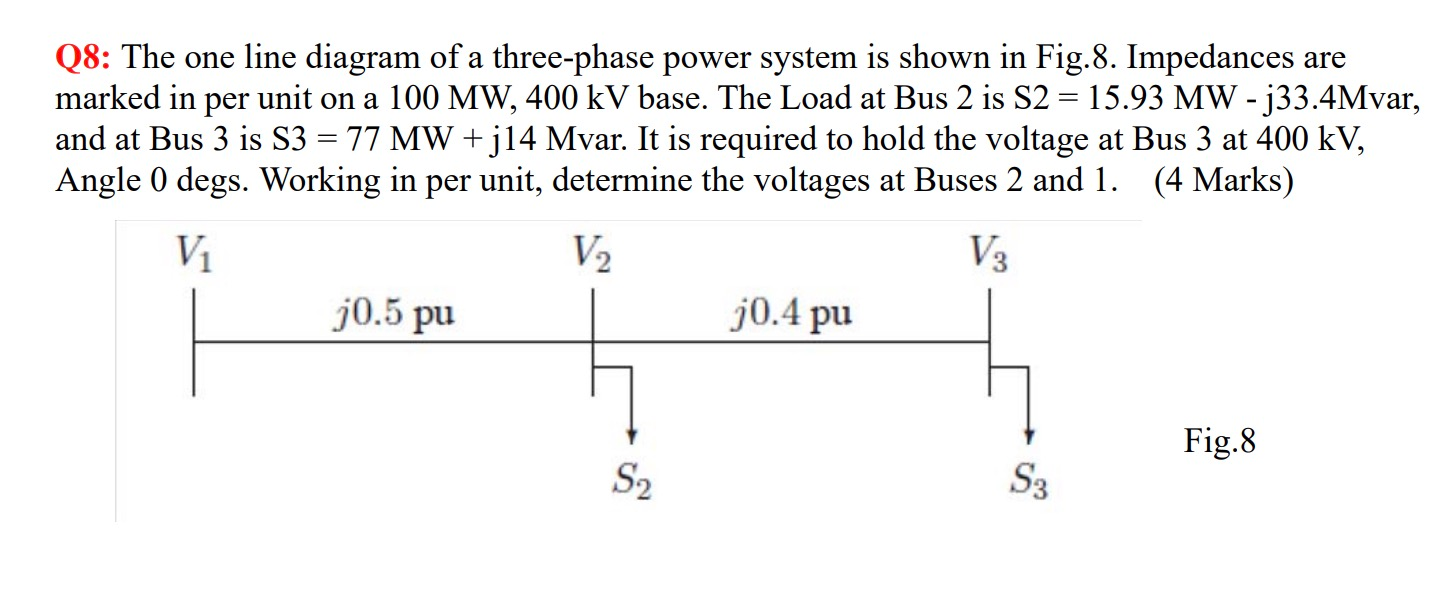The one line diagram of a three-phase power system is shown in Fig.8. Impedances are marked in per unit on a 100 MW, 400 kV base. The Load at Bus 2 is S2 = 15.93 MW - j33.4Mvar, and at Bus 3 is S3 = 77 MW + j14 Mvar. It is required to hold the voltage at Bus 3 at 400 kV, Angle 0 degs. Working in per unit, determine the voltages at Buses 2 and 1. Q8: The...

• ### The one-line diagram of a simple power system is shown in Figure 1. The neutral of...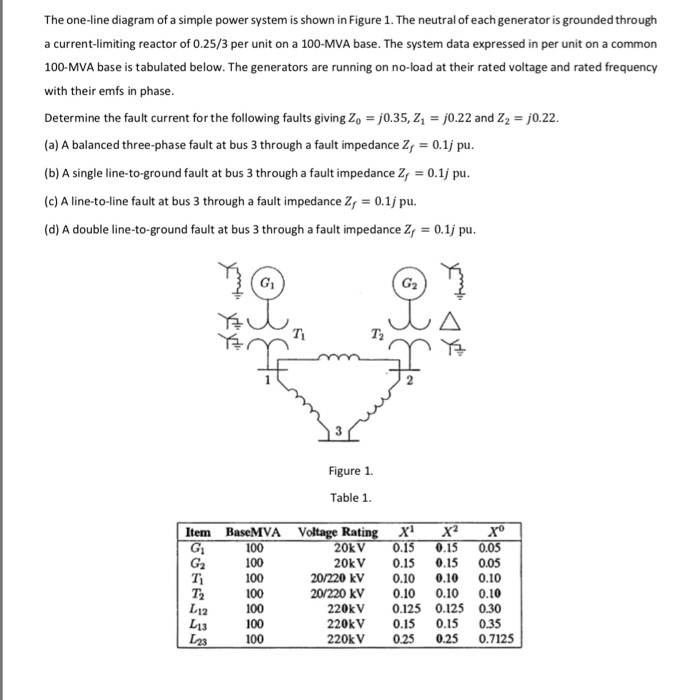The one-line diagram of a simple power system is shown in Figure 1. The neutral of each generator is grounded through a current-limiting reactor of 0.25/3 per unit on a 100-MVA base. The system data expressed in per unit on a common 100-MVA base is tabulated below. The generators are running on no-load at their rated voltage and rated frequency with their emfs in phase. Determine the fault current for the following faults giving Zo = 0.35, Z = 0.22...

• ### 2. A single-line diagram of the power system considered is shown in Figure P2a, where negative-...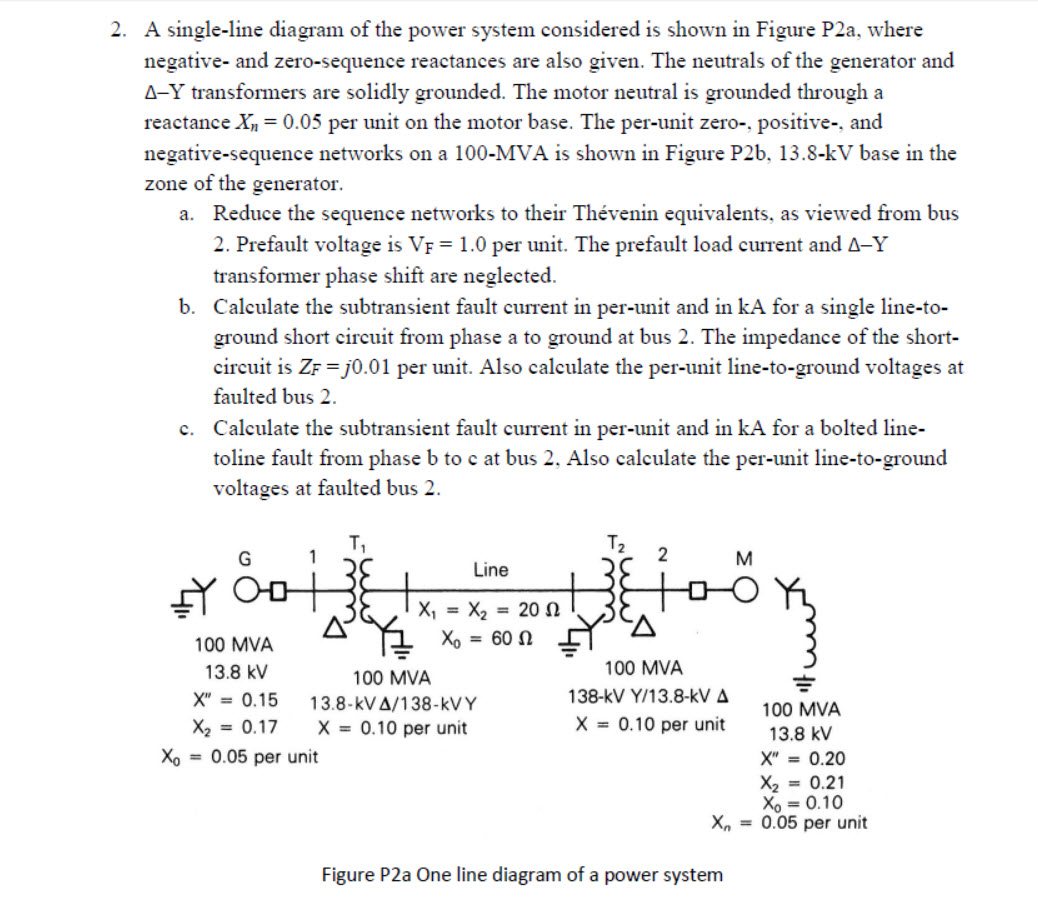2. A single-line diagram of the power system considered is shown in Figure P2a, where negative- and zero-sequence reactances are also given. The neutrals of the generator and A-Y transformers are solidly grounded. The motor neutral is grounded through a reactance Xn = 0.05 per unit on the motor base. The per-unit zero-, positive and negative-sequence networks on a 100-MVA is shown in Figure P26, 13.8-kV base in the zone of the generator. a. Reduce the sequence networks to their...

• ### A 7-bus power system with three generators, six transformers, and seven transmission lines is shown in...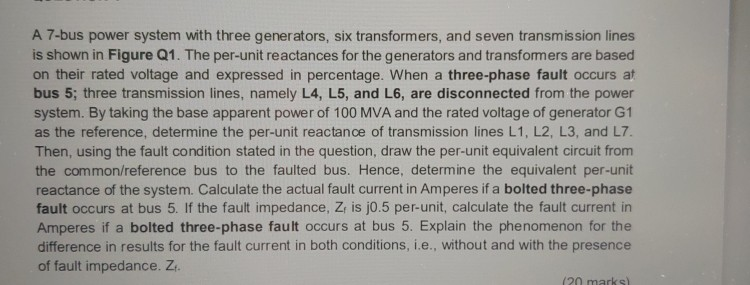A 7-bus power system with three generators, six transformers, and seven transmission lines is shown in Figure Q1. The per-unit reactances for the generators and transfomers are based on their rated voltage and expressed in percentage. When a three-phase fault occurs at bus 5; three transmission lines, namely L4, L5, and L6, are disconnected from the power system. By taking the base apparent power of 100 MVA and the rated voltage of generator G1 as the reference, determine the per-unit...

• ### The reactance data for the power system shown in figure 10.30 in per unit on a...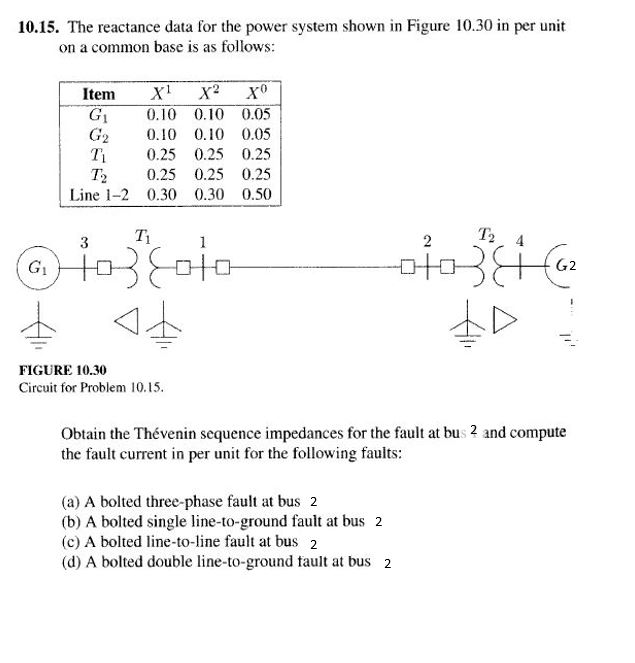The reactance data for the power system shown in figure 10.30 in per unit on a common base is as follows: *************Remember calculations are needed at bus 2 **************** 10.15. The reactance data for the power system shown in Figure 10.30 in per unit on a common base is as follows Item X1 G1 0.10 0.10 0.05 G2 0.10 0.10 0.05 T, 0.25 0.25 0.25 T 0.25 0.25 0.25 Line 1-2 0.30 0.30 0.50 Ti 1 3 2 FIGURE 10.30...

• ### Power system operation and control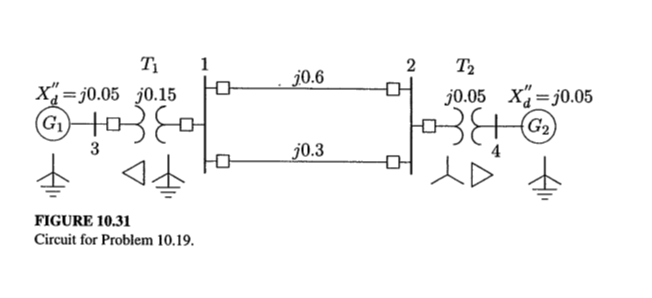The positive-sequence reactances for the power system shown in Figure 10.31 are in per unit on a common MVA base. Resistances are neglected and the negative-sequence impedances are assumed to be the same as the positive-sequence impedances. A bolted line-to-line fault occurs between phases b and c at bus 2.Before the fault occurrence, all bus voltages are 1.0 per unit. Obtain the positive-sequence bus impedance matrix.Find the fault current, the three-phase bus voltages during fault, and the line currents in...

• ### symmetrical three phase fault at bus 4 the fault current the bus voltages all the line...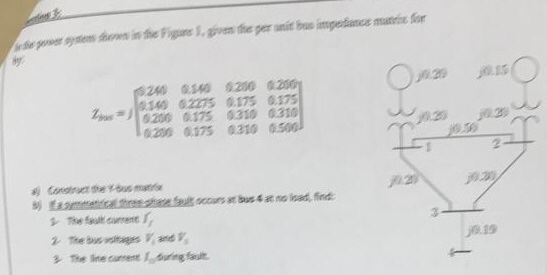symmetrical three phase fault at bus 4 the fault current the bus voltages all the line currents all find y bus ysy ews in fhe Figune 1,gven the per unit bus impedance matri 20 240 0140 0200 0200 a140 02275 0175 0175 Zp 200 G175 0310 0310 0200 0175 0310 0500 20 050 N2 Condinut the yusmai hee feut sccurs at bus 4 at no lead, find The fault current 2 The bus tages and 3The line carent 1during fault...

• ### Busl Bus2 Bus3 Figure 1: One line diagram All impedances are specified in per unit. For...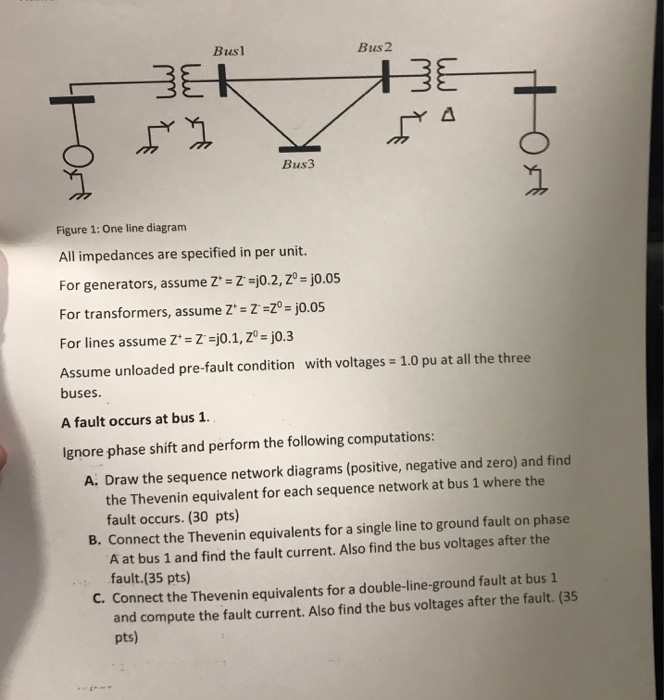Busl Bus2 Bus3 Figure 1: One line diagram All impedances are specified in per unit. For generators, assume Z'- Zj0.2,Z- j0.05 For transformers, assume Z -Z -Zo - j0.05 For lines assume Z+= Z'=j0.1,20-jo.3 Assume unloaded pre-fault condition with voltages 1.0 pu at all the three buses. A fault occurs at bus 1. Ignore phase shift and perform the following computations: A. Draw the sequence network diagrams (positive, negative and zero) and find the Thevenin equivalent for each sequence network...

• ### A single line diagram of a power system is shown in Fig. 2. The system data with equipment ratings and assumed sequence reactances are given the following table. The neutrals of the generator and A-Y...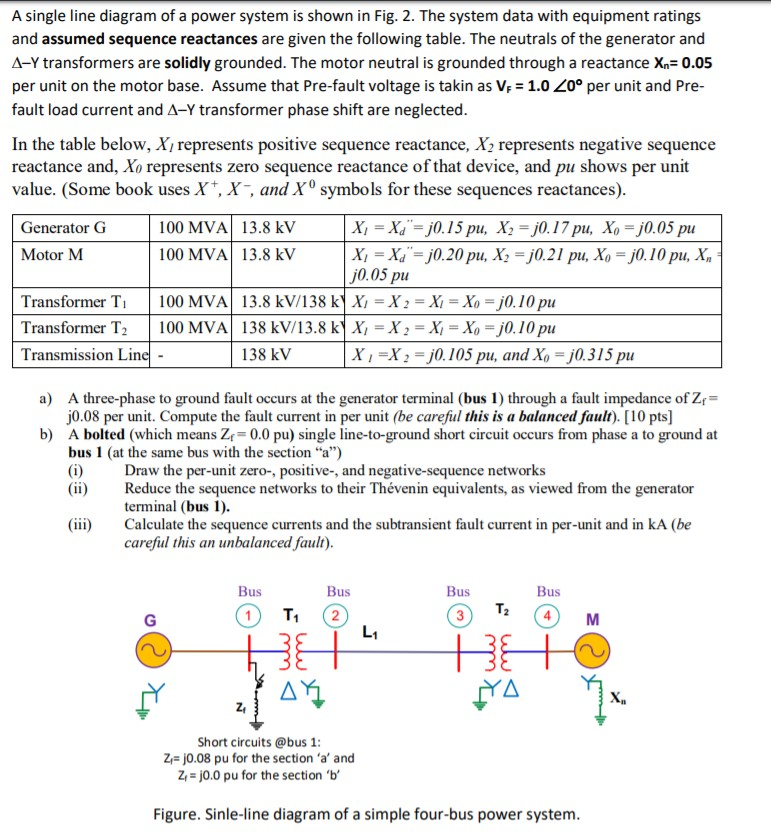A single line diagram of a power system is shown in Fig. 2. The system data with equipment ratings and assumed sequence reactances are given the following table. The neutrals of the generator and A-Y transformers are solidly grounded. The motor neutral is grounded through a reactance Xn 0.05 per unit on the motor base. Assume that Pre-fault voltage is takin as VF-1.0 ,0° per unit and Pre- fault load current and Δ-Y transformer phase shift are neglected In the...

• ### QUESTION 4. A single-line diagram of a power system is shown in Figure Q3 below, where...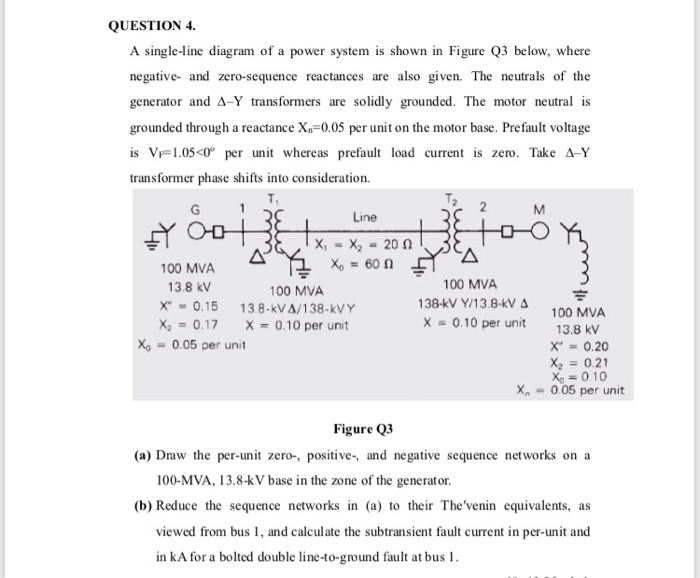QUESTION 4. A single-line diagram of a power system is shown in Figure Q3 below, where negative and zero-sequence reactances are also given. The neutrals of the generator and A-Y transformers are solidly grounded. The motor neutral is grounded through a reactance X.=0.05 per unit on the motor base. Prefault voltage is VF1.05<Oº per unit whereas prefault load current is zero. Take A-Y transformer phase shifts into consideration. M Line tool X, - X2 - 200 100 MVA X =...